# Mean State

Period Mean (original grids) [W/m2]
Model Period Mean (intersection) [W/m2]
Model Period Mean (complement) [W/m2]
Benchmark Period Mean (intersection) [W/m2]
Benchmark Period Mean (complement) [W/m2]
Bias [W/m2]
RMSE [W/m2]
Phase Shift [months]
Bias Score 
RMSE Score 
Seasonal Cycle Score 
Spatial Distribution Score 
Interannual Variability Score 
Overall Score 
Benchmark [-] 187.
CRUNCEPv7 [-] 192. 192. 186. 206. 5.63 16.6 1.10 0.616 0.486 0.858 0.955 0.476 0.646
GSWP3v1 [-] 182. 182. 186. 206. -4.31 15.3 0.775 0.727 0.494 0.907 0.924 0.800 0.724
WFDEI [-] 167. 167. 186. 206. -18.6 28.6 1.40 0.388 0.374 0.799 0.952 0.776 0.611
Period Mean (original grids) [W/m2]
Model Period Mean (intersection) [W/m2]
Model Period Mean (complement) [W/m2]
Benchmark Period Mean (intersection) [W/m2]
Benchmark Period Mean (complement) [W/m2]
Bias [W/m2]
RMSE [W/m2]
Phase Shift [months]
Bias Score 
RMSE Score 
Seasonal Cycle Score 
Spatial Distribution Score 
Interannual Variability Score 
Overall Score 
Benchmark [-] 198.
CRUNCEPv7 [-] 198. 198. 198. 214. 0.100 18.7 1.51 0.569 0.472 0.781 0.931 0.725 0.658
GSWP3v1 [-] 194. 194. 198. 214. -3.39 14.2 0.702 0.718 0.513 0.923 0.979 0.833 0.747
WFDEI [-] 190. 190. 198. 214. -7.32 20.3 0.916 0.553 0.454 0.895 0.995 0.809 0.693
Period Mean (original grids) [W/m2]
Model Period Mean (intersection) [W/m2]
Model Period Mean (complement) [W/m2]
Benchmark Period Mean (intersection) [W/m2]
Benchmark Period Mean (complement) [W/m2]
Bias [W/m2]
RMSE [W/m2]
Phase Shift [months]
Bias Score 
RMSE Score 
Seasonal Cycle Score 
Spatial Distribution Score 
Interannual Variability Score 
Overall Score 
Benchmark [-] 199.
CRUNCEPv7 [-] 195. 195. 198. 219. -2.87 17.1 1.13 0.713 0.557 0.837 0.850 0.527 0.674
GSWP3v1 [-] 196. 197. 198. 219. -2.35 15.5 0.774 0.801 0.568 0.893 0.995 0.744 0.762
WFDEI [-] 196. 196. 198. 219. -2.31 21.1 1.13 0.715 0.470 0.832 0.988 0.730 0.701
Period Mean (original grids) [W/m2]
Model Period Mean (intersection) [W/m2]
Model Period Mean (complement) [W/m2]
Benchmark Period Mean (intersection) [W/m2]
Benchmark Period Mean (complement) [W/m2]
Bias [W/m2]
RMSE [W/m2]
Phase Shift [months]
Bias Score 
RMSE Score 
Seasonal Cycle Score 
Spatial Distribution Score 
Interannual Variability Score 
Overall Score 
Benchmark [-] 70.7
CRUNCEPv7 [-] 72.8 72.9 70.5 72.8 1.13 20.4 0.333 0.910 0.748 0.978 0.995 0.710 0.848
GSWP3v1 [-] 70.3 70.4 70.5 72.8 -0.648 18.5 0.319 0.934 0.761 0.979 0.998 0.763 0.866
WFDEI [-] 60.0 60.2 70.5 72.8 -10.7 23.4 0.382 0.849 0.732 0.974 0.987 0.845 0.853
Period Mean (original grids) [W/m2]
Model Period Mean (intersection) [W/m2]
Model Period Mean (complement) [W/m2]
Benchmark Period Mean (intersection) [W/m2]
Benchmark Period Mean (complement) [W/m2]
Bias [W/m2]
RMSE [W/m2]
Phase Shift [months]
Bias Score 
RMSE Score 
Seasonal Cycle Score 
Spatial Distribution Score 
Interannual Variability Score 
Overall Score 
Benchmark [-] 194.
CRUNCEPv7 [-] 187. 187. 190. 207. -0.526 18.0 1.52 0.610 0.443 0.765 0.843 0.519 0.604
GSWP3v1 [-] 176. 176. 190. 207. -10.3 18.7 1.27 0.569 0.436 0.800 0.963 0.769 0.662
WFDEI [-] 165. 165. 190. 207. -21.0 28.1 1.67 0.362 0.390 0.742 0.991 0.686 0.593
Period Mean (original grids) [W/m2]
Model Period Mean (intersection) [W/m2]
Model Period Mean (complement) [W/m2]
Benchmark Period Mean (intersection) [W/m2]
Benchmark Period Mean (complement) [W/m2]
Bias [W/m2]
RMSE [W/m2]
Phase Shift [months]
Bias Score 
RMSE Score 
Seasonal Cycle Score 
Spatial Distribution Score 
Interannual Variability Score 
Overall Score 
Benchmark [-] 97.8
CRUNCEPv7 [-] 109. 109. 97.6 118. 11.2 20.9 0.260 0.845 0.794 0.983 0.995 0.716 0.854
GSWP3v1 [-] 102. 102. 97.6 118. 4.51 14.6 0.227 0.926 0.827 0.985 0.995 0.668 0.871
WFDEI [-] 94.7 94.7 97.6 118. -2.51 18.3 0.358 0.934 0.772 0.976 0.984 0.798 0.873
Period Mean (original grids) [W/m2]
Model Period Mean (intersection) [W/m2]
Model Period Mean (complement) [W/m2]
Benchmark Period Mean (intersection) [W/m2]
Benchmark Period Mean (complement) [W/m2]
Bias [W/m2]
RMSE [W/m2]
Phase Shift [months]
Bias Score 
RMSE Score 
Seasonal Cycle Score 
Spatial Distribution Score 
Interannual Variability Score 
Overall Score 
Benchmark [-] 139.
CRUNCEPv7 [-] 149. 149. 138. 149. 11.1 23.8 0.446 0.752 0.744 0.960 0.973 0.716 0.815
GSWP3v1 [-] 136. 136. 138. 149. -2.20 15.9 0.348 0.880 0.767 0.970 0.999 0.670 0.842
WFDEI [-] 142. 141. 138. 149. 3.22 23.6 0.527 0.826 0.677 0.951 0.950 0.770 0.808
Period Mean (original grids) [W/m2]
Model Period Mean (intersection) [W/m2]
Model Period Mean (complement) [W/m2]
Benchmark Period Mean (intersection) [W/m2]
Benchmark Period Mean (complement) [W/m2]
Bias [W/m2]
RMSE [W/m2]
Phase Shift [months]
Bias Score 
RMSE Score 
Seasonal Cycle Score 
Spatial Distribution Score 
Interannual Variability Score 
Overall Score 
Benchmark [-] 181.
CRUNCEPv7 [-] 194. 194. 179. 205. 14.7 26.0 0.341 0.568 0.584 0.973 0.964 0.652 0.721
GSWP3v1 [-] 175. 175. 179. 205. -3.00 18.1 0.342 0.776 0.609 0.973 0.946 0.723 0.772
WFDEI [-] 175. 175. 179. 205. -3.64 24.6 0.502 0.688 0.518 0.959 0.998 0.711 0.732
Period Mean (original grids) [W/m2]
Model Period Mean (intersection) [W/m2]
Model Period Mean (complement) [W/m2]
Benchmark Period Mean (intersection) [W/m2]
Benchmark Period Mean (complement) [W/m2]
Bias [W/m2]
RMSE [W/m2]
Phase Shift [months]
Bias Score 
RMSE Score 
Seasonal Cycle Score 
Spatial Distribution Score 
Interannual Variability Score 
Overall Score 
Benchmark [-] 62.8
CRUNCEPv7 [-] 64.2 64.7 63.2 54.4 0.281 20.6 0.281 0.924 0.732 0.981 0.960 0.754 0.847
GSWP3v1 [-] 61.3 61.7 63.2 54.4 -2.47 16.4 0.176 0.941 0.777 0.988 0.984 0.759 0.871
WFDEI [-] 50.5 51.0 63.2 54.4 -13.1 24.3 0.308 0.815 0.721 0.980 0.993 0.848 0.846
Period Mean (original grids) [W/m2]
Model Period Mean (intersection) [W/m2]
Model Period Mean (complement) [W/m2]
Benchmark Period Mean (intersection) [W/m2]
Benchmark Period Mean (complement) [W/m2]
Bias [W/m2]
RMSE [W/m2]
Phase Shift [months]
Bias Score 
RMSE Score 
Seasonal Cycle Score 
Spatial Distribution Score 
Interannual Variability Score 
Overall Score 
Benchmark [-] 198.
CRUNCEPv7 [-] 194. 194. 198. 197. -5.32 15.0 0.501 0.837 0.776 0.966 0.967 0.650 0.829
GSWP3v1 [-] 190. 190. 198. 197. -9.01 16.6 0.371 0.822 0.764 0.971 1.00 0.706 0.838
WFDEI [-] 194. 194. 198. 197. -5.97 19.9 0.422 0.862 0.682 0.969 0.998 0.810 0.834
Period Mean (original grids) [W/m2]
Model Period Mean (intersection) [W/m2]
Model Period Mean (complement) [W/m2]
Benchmark Period Mean (intersection) [W/m2]
Benchmark Period Mean (complement) [W/m2]
Bias [W/m2]
RMSE [W/m2]
Phase Shift [months]
Bias Score 
RMSE Score 
Seasonal Cycle Score 
Spatial Distribution Score 
Interannual Variability Score 
Overall Score 
Benchmark [-] 120.
CRUNCEPv7 [-] 133. 133. 118. 145. 15.5 24.9 0.231 0.764 0.800 0.985 0.995 0.732 0.846
GSWP3v1 [-] 114. 114. 118. 145. -1.47 13.2 0.209 0.926 0.841 0.986 0.993 0.658 0.874
WFDEI [-] 113. 113. 118. 145. -2.40 20.1 0.364 0.911 0.759 0.976 0.995 0.826 0.871
Period Mean (original grids) [W/m2]
Model Period Mean (intersection) [W/m2]
Model Period Mean (complement) [W/m2]
Benchmark Period Mean (intersection) [W/m2]
Benchmark Period Mean (complement) [W/m2]
Bias [W/m2]
RMSE [W/m2]
Phase Shift [months]
Bias Score 
RMSE Score 
Seasonal Cycle Score 
Spatial Distribution Score 
Interannual Variability Score 
Overall Score 
Benchmark [-] 163.
CRUNCEPv7 [-] 144. 144. 142. 173. 1.83 19.8 0.580 0.746 0.685 0.934 0.994 0.613 0.776
GSWP3v1 [-] 137. 137. 142. 173. -4.46 16.0 0.389 0.822 0.705 0.959 0.998 0.712 0.817
WFDEI [-] 136. 136. 142. 173. -5.53 21.5 0.584 0.753 0.638 0.934 0.996 0.747 0.784
Period Mean (original grids) [W/m2]
Model Period Mean (intersection) [W/m2]
Model Period Mean (complement) [W/m2]
Benchmark Period Mean (intersection) [W/m2]
Benchmark Period Mean (complement) [W/m2]
Bias [W/m2]
RMSE [W/m2]
Phase Shift [months]
Bias Score 
RMSE Score 
Seasonal Cycle Score 
Spatial Distribution Score 
Interannual Variability Score 
Overall Score 
Benchmark [-] 177.
CRUNCEPv7 [-] 168. 168. 175. 217. -6.22 17.9 0.405 0.721 0.749 0.965 0.985 0.709 0.813
GSWP3v1 [-] 162. 162. 175. 217. -12.0 17.9 0.298 0.709 0.763 0.978 0.963 0.673 0.808
WFDEI [-] 172. 172. 175. 217. -2.53 16.8 0.507 0.777 0.731 0.955 0.976 0.652 0.804
Period Mean (original grids) [W/m2]
Model Period Mean (intersection) [W/m2]
Model Period Mean (complement) [W/m2]
Benchmark Period Mean (intersection) [W/m2]
Benchmark Period Mean (complement) [W/m2]
Bias [W/m2]
RMSE [W/m2]
Phase Shift [months]
Bias Score 
RMSE Score 
Seasonal Cycle Score 
Spatial Distribution Score 
Interannual Variability Score 
Overall Score 
Benchmark [-] 69.7
CRUNCEPv7 [-] 74.1 74.0 69.6 71.1 2.14 20.6 0.113 0.891 0.751 0.992 0.928 0.621 0.822
GSWP3v1 [-] 69.2 69.2 69.6 71.1 -1.83 17.2 0.0813 0.920 0.779 0.995 0.989 0.765 0.871
WFDEI [-] 62.0 61.9 69.6 71.1 -9.33 23.4 0.141 0.852 0.726 0.991 0.946 0.799 0.840
Period Mean (original grids) [W/m2]
Model Period Mean (intersection) [W/m2]
Model Period Mean (complement) [W/m2]
Benchmark Period Mean (intersection) [W/m2]
Benchmark Period Mean (complement) [W/m2]
Bias [W/m2]
RMSE [W/m2]
Phase Shift [months]
Bias Score 
RMSE Score 
Seasonal Cycle Score 
Spatial Distribution Score 
Interannual Variability Score 
Overall Score 
Benchmark [-] 204.
CRUNCEPv7 [-] 190. 190. 201. 219. -8.69 24.0 0.969 0.610 0.645 0.900 0.820 0.666 0.715
GSWP3v1 [-] 192. 192. 201. 219. -6.68 16.7 0.458 0.769 0.678 0.945 0.986 0.753 0.801
WFDEI [-] 194. 194. 201. 219. -6.90 21.4 0.489 0.721 0.614 0.938 0.997 0.703 0.764
Period Mean (original grids) [W/m2]
Model Period Mean (intersection) [W/m2]
Model Period Mean (complement) [W/m2]
Benchmark Period Mean (intersection) [W/m2]
Benchmark Period Mean (complement) [W/m2]
Bias [W/m2]
RMSE [W/m2]
Phase Shift [months]
Bias Score 
RMSE Score 
Seasonal Cycle Score 
Spatial Distribution Score 
Interannual Variability Score 
Overall Score 
Benchmark [-] 150.
CRUNCEPv7 [-] 155. 156. 148. 192. 8.22 18.2 0.417 0.833 0.783 0.972 0.986 0.577 0.822
GSWP3v1 [-] 145. 145. 148. 192. -1.45 13.3 0.293 0.939 0.801 0.981 0.991 0.701 0.869
WFDEI [-] 150. 150. 148. 192. 3.00 22.3 0.394 0.903 0.690 0.974 0.998 0.838 0.849
Period Mean (original grids) [W/m2]
Model Period Mean (intersection) [W/m2]
Model Period Mean (complement) [W/m2]
Benchmark Period Mean (intersection) [W/m2]
Benchmark Period Mean (complement) [W/m2]
Bias [W/m2]
RMSE [W/m2]
Phase Shift [months]
Bias Score 
RMSE Score 
Seasonal Cycle Score 
Spatial Distribution Score 
Interannual Variability Score 
Overall Score 
Benchmark [-] 110.
CRUNCEPv7 [-] 122. 123. 110. 117. 12.7 21.9 0.218 0.827 0.797 0.986 0.971 0.562 0.823
GSWP3v1 [-] 112. 112. 110. 117. 1.79 13.4 0.112 0.929 0.841 0.993 0.999 0.675 0.880
WFDEI [-] 111. 111. 110. 117. 1.60 19.6 0.186 0.914 0.770 0.988 0.941 0.840 0.870
Period Mean (original grids) [W/m2]
Model Period Mean (intersection) [W/m2]
Model Period Mean (complement) [W/m2]
Benchmark Period Mean (intersection) [W/m2]
Benchmark Period Mean (complement) [W/m2]
Bias [W/m2]
RMSE [W/m2]
Phase Shift [months]
Bias Score 
RMSE Score 
Seasonal Cycle Score 
Spatial Distribution Score 
Interannual Variability Score 
Overall Score 
Benchmark [-] 161.
CRUNCEPv7 [-] 155. 155. 161. 163. -5.79 16.7 0.204 0.885 0.813 0.986 0.938 0.611 0.841
GSWP3v1 [-] 160. 160. 161. 163. -0.462 14.3 0.198 0.928 0.826 0.987 0.983 0.702 0.875
WFDEI [-] 169. 169. 161. 163. 8.11 23.2 0.247 0.880 0.739 0.983 0.998 0.838 0.863
Period Mean (original grids) [W/m2]
Model Period Mean (intersection) [W/m2]
Model Period Mean (complement) [W/m2]
Benchmark Period Mean (intersection) [W/m2]
Benchmark Period Mean (complement) [W/m2]
Bias [W/m2]
RMSE [W/m2]
Phase Shift [months]
Bias Score 
RMSE Score 
Seasonal Cycle Score 
Spatial Distribution Score 
Interannual Variability Score 
Overall Score 
Benchmark [-] 179.
CRUNCEPv7 [-] 183. 183. 178. 218. 6.21 17.3 1.49 0.601 0.451 0.776 0.822 0.578 0.613
GSWP3v1 [-] 174. 174. 178. 218. -3.01 14.3 0.799 0.751 0.449 0.896 0.959 0.806 0.718
WFDEI [-] 160. 160. 178. 218. -17.7 27.4 1.47 0.351 0.353 0.799 0.994 0.639 0.581
Period Mean (original grids) [W/m2]
Model Period Mean (intersection) [W/m2]
Model Period Mean (complement) [W/m2]
Benchmark Period Mean (intersection) [W/m2]
Benchmark Period Mean (complement) [W/m2]
Bias [W/m2]
RMSE [W/m2]
Phase Shift [months]
Bias Score 
RMSE Score 
Seasonal Cycle Score 
Spatial Distribution Score 
Interannual Variability Score 
Overall Score 
Benchmark [-] 185.
CRUNCEPv7 [-] 187. 187. 184. 202. 2.66 20.0 0.995 0.699 0.619 0.884 0.821 0.518 0.693
GSWP3v1 [-] 180. 181. 184. 202. -3.37 15.5 0.717 0.831 0.645 0.909 0.976 0.715 0.787
WFDEI [-] 175. 175. 184. 202. -8.85 23.8 1.03 0.709 0.529 0.865 0.958 0.779 0.728
Period Mean (original grids) [W/m2]
Model Period Mean (intersection) [W/m2]
Model Period Mean (complement) [W/m2]
Benchmark Period Mean (intersection) [W/m2]
Benchmark Period Mean (complement) [W/m2]
Bias [W/m2]
RMSE [W/m2]
Phase Shift [months]
Bias Score 
RMSE Score 
Seasonal Cycle Score 
Spatial Distribution Score 
Interannual Variability Score 
Overall Score 
Benchmark [-] 178.
CRUNCEPv7 [-] 179. 178. 179. 169. -3.51 27.2 0.548 0.689 0.688 0.947 0.804 0.632 0.741
GSWP3v1 [-] 177. 176. 179. 169. -7.13 20.8 0.400 0.791 0.712 0.959 0.998 0.687 0.810
WFDEI [-] 182. 181. 179. 169. -2.00 24.2 0.510 0.771 0.675 0.945 0.988 0.719 0.796

# Temporally integrated period mean

BENCHMARK MEAN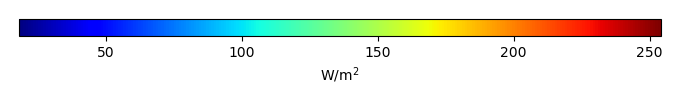MODEL MEANBIAS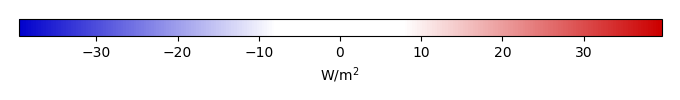BIAS SCORERMSE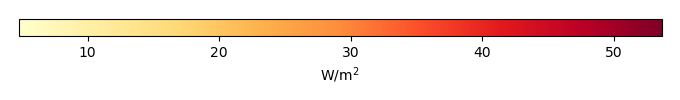RMSE SCOREBENCHMARK INTERANNUAL VARIABILITY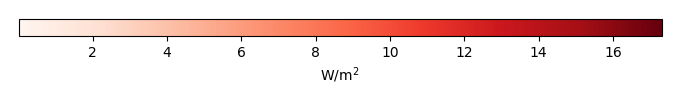MODEL INTERANNUAL VARIABILITYINTERANNUAL VARIABILITY SCOREBENCHMARK MAX MONTHMODEL MAX MONTHDIFFERENCE IN MAX MONTH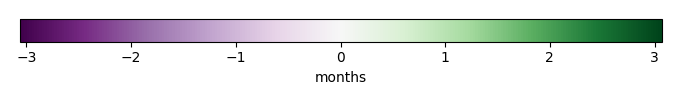SEASONAL CYCLE SCORESPATIAL TAYLOR DIAGRAMMODEL COLORS# Spatially integrated regional mean

MODEL COLORSREGIONAL MEANANNUAL CYCLEMONTHLY ANOMALYANNUAL CYCLE# All Models

BenchmarkCRUNCEPv7GSWP3v1WFDEI# Data Information

creation_date: Thu May 8 23:03:44 PDT 2014

source_file: This product is generated from monthly 1 degree CERES EBAF Radiation observations